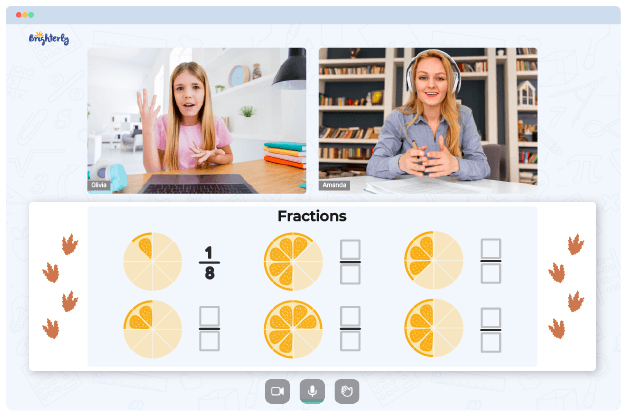The 4th grade is a pivotal period for any young learner because they get introduced to a wide range of math concepts such as fractions. In this grade, students learn about different types of fractions and how to solve them. This new information can be overwhelming and difficult to process. This is where our 4th grade fractions worksheets come in. This educational guide covers all aspects of fractions, equipping students with both theoretical and practical knowledge.

Leave your email and we will send you worksheets

worksheets sent successfully

These worksheets introduce young learners to basic concepts through practice questions. In the 4th grade math worksheets fractions, kids learn how to identify unit fractions and mixed numbers. But it doesn’t just end there. They also learn how to differentiate between proper and improper fractions by differentiating between the numerator and the denominator.

Math for Kids

Is Your Child Struggling With Math?
1:1 Online Math TutoringAfter using these 4th grade fraction worksheets, young learners will be equipped with enhanced recognition, simplifying, and differentiation skills. They’ll also become better in other areas such as multiplication and complex division.

Leave your email and we will send you worksheets

worksheets sent successfully### Fraction Worksheets 4Th Grade PDF### Fraction Worksheets 4Th Grade PDF### Fraction Worksheets 4Th Grade PDF### Fraction Worksheets 4Th Grade PDF

These worksheets are a great addition to any student’s arsenal because they cover all the bases; from adding and subtracting fractions to conversions, and so on. More importantly, the questions are presented in an easily comprehensible layout, which makes the tasks less frustrating for the young learner.

### More Fractions Worksheets

Troubles with Fractions?• Does your child need extra help with understanding the concept of fractions?
• Start studying with an online tutor.

Is your child finding it hard to master fractions concepts? An online tutor could be of assistance.